Published: 09 October 2016

# The NAH based on complex cepstrum method in a closed space

Xianglin Tian1
Jingjun Lou2
Yangyang Zhang3
Duo Qu4
1, 3, 4College of Power Engineering, Naval University of Engineering, Wuhan 430033, China
2Scientific Research Ministry of Naval University of Engineering, Wuhan 430033, China
Corresponding Author:
Xianglin Tian
Views 20

#### Abstract

NAH method in non-free sound field reconstruction of the sound source will lead to considerable error. This paper proposes a method to reconstruct the sound source in a closed space. In the closed space, the total sound pressure is the sum of the source radiation pressure and the reflected sound pressure from medium interface. Reflections from medium interface is a convolution noise, rather than additive noise. In order to reconstruct a sound source in the closed space, in this paper we first adopt the complex cepstrum method to separate and radiation pressure and the reflected sound pressure, then filter them to reduction of reflected sound pressure, and then reconstruct the sound source. The simulation results show the correctness and effectiveness of the method.

## 1. Introduction

In the early 1980s, american scholar E. G Williams put forward the concept [1, 2]. NAH considering the effect of evanescent waves, breaking the Rayleigh criterion limiting the resolution of reconstruction in recent years has been widely used. NAH is different from of the far field acoustic holography. In the holographic surface near the sound source surface, the measurement data is recorded. The sound pressure is then reconstruct three-dimensional sound field, sound intensity, and particle velocity by the Fourier technology. Since the limit of the two

-dimensional Fourier transform itself, planar NAH required the measured surface should be conformal of the sound source , in order to make NAH can be applied to any surface shape of the sound source, the boundary element method  have been proposed. Xinzhao Chen propose the distributed source boundary point method , further simplifying the tedious calculations boundary element method for the coefficient matrix. In order to improve the accuracy of the reconstruction effectively, many scholars in-depth study of the data interpolation and extrapolation methods [6-10].

Planar NAH basing on spatial sound field transformation requires sound field to be free-field measurements, which severely limits the planar NAH applications. Dual holographic  located on different sides of the holographic interference source separation plane NAH further expand its application, but the application plane NAH reconstruction of the sound source in an enclosed space will still produce large errors. In this paper, the complex cepstrum preprocess the measured data before reconstruction, to eliminate or weaken the effects of reflected sound.

## 2. The theory of the planar acoustic holography

The acoustic wave propagation satisfies the equation  in the three-dimensional ideal fluid medium:

1
${\rho }_{0}\frac{\partial \stackrel{\to }{v}\left(r,t\right)}{\partial t}=-\nabla p\left(r,t\right),\mathrm{}\mathrm{}\mathrm{}\mathrm{}-{\rho }_{0}div\stackrel{\to }{v}\left(r,t\right)=\frac{\partial {\rho }^{\mathrm{\text{'}}}\left(r,t\right)}{\partial t},p\left(r,t\right)={c}^{2}{\rho }^{\mathrm{\text{'}}}\left(r,t\right).$

The solutions of the above three equations is:

2
${\nabla }^{2}p\left(x,y,z,t\right)=\frac{1}{{c}_{0}^{2}}\frac{{\partial }^{2}p\left(x,y,z,t\right)}{{\partial }^{2}t}.$

Fourier transform on both sides, we can obtain:

3
${\nabla }^{2}p\left(x,y,z\right)+{k}^{2}p\left(x,y,z\right)=0,$

where $p\left(x,y,z\right)$ is the frequency domain pressure; $k=\omega /c$ is the wave number; $\omega$ is the circular frequency; $c$ is the speed of sound.

The formula is Helmhotz equation, the equation always hold to steady sound. The solution of Eq. (3) can be expressed as the Kirchhoff integral:

4
$p\left(x,y,z\right)=\underset{S}{\iint }\left[g\left(r,{r}_{S}\right)\frac{\partial p\left(S\right)}{\partial n}-p\left(S\right)\frac{\partial g\left(r,{r}_{S}\right)}{\partial n}\right]ds,$

where $s$ is a closed surface, $r$ is the distance from the point to the origin from the field, ${r}_{S}$ is the distance from the sound source surface to the point in the sound field, $p\left(S\right)$ is the complex sound pressure, $g\left(r,{r}_{S}\right)$ is Green’s function.

In an infinite radius hemispherical surface of the sound field, Eq. (4) can be expressed as:

5
$p\left(x,y,z\right)=\underset{{S}_{zs}}{\iint }\left[g\left(r,{r}_{S}\right)\frac{\partial p\left(S\right)}{\partial n}-p\left(S\right)\frac{\partial g\left(r,{r}_{S}\right)}{\partial n}\right]ds$
$\mathrm{}\mathrm{}\mathrm{}\mathrm{}\mathrm{}\mathrm{}+\underset{{S}_{\mathrm{\infty }}}{\iint }\left[g\left(r,{r}_{S}\right)\frac{\partial p\left(S\right)}{\partial n}-p\left(S\right)\frac{\partial g\left(r,{r}_{S}\right)}{\partial n}\right]ds.$

Semi-free sound field infinity meet sommerfeld radiation conditions, Eq. (5) in the second integral is zero, so Eq. (5) simplifies to:

6
$p\left(x,y,z\right)=\underset{{S}_{zs}}{\iint }\left[g\left(r,{r}_{S}\right)\frac{\partial p\left(S\right)}{\partial n}-p\left(S\right)\frac{\partial g\left(r,{r}_{S}\right)}{\partial n}\right]ds.$

In actual sound field, the sound pressure distribution satisfies Dirichlet conditions, the Green’s function is:

7
$\left\{\begin{array}{l}g\left(r,{r}_{S}\right){|}_{{r}_{S}}=0,\\ -\frac{\partial g\left(r,{r}_{S}\right)}{\partial n}={g}_{D}\left(r,{r}_{S}\right).\end{array}\right\$

Meet the conditions for the Green’s function expression:

8
${g}_{D}\left(r,{r}_{S}\right)=\frac{z\left(1-ik{r}^{\text{'}}\right){e}^{ikr}}{2\pi {r}^{\text{'}3}},$

where ${r}^{\text{'}}$ is the distance from the sound source surface, Eq. (6) simplifies to:

9
$p\left(r\right)=\underset{{S}_{zs}}{\iint }p\left(S\right){g}_{D}\left(r,{r}_{S}\right)ds.$

In the Cartesian coordinate system, Eq. (9) can be express as:

10
$p\left(x,y,z\right)=\underset{{S}_{zs}}{\iint }p\left({x}_{zs},{y}_{zs},{z}_{zs}\right){g}_{D}\left(x-{x}_{zs},y-{y}_{zs},z-{z}_{zs}\right)ds.$

According to the convolution theorem there is:

11
$\stackrel{^}{P}\left({k}_{x},{k}_{y},z\right)=\stackrel{^}{P}\left({k}_{x},{k}_{y},{z}_{s}\right){\stackrel{^}{G}}_{D}\left({k}_{x},{k}_{y},z-{z}_{s}\right)$

where:

12
${\stackrel{^}{G}}_{D}\left({k}_{x},{k}_{y},z-{z}_{s}\right)=\left\{\begin{array}{l}\mathrm{exp}\left(i\left(z-{z}_{s}\right)\sqrt{{k}^{2}-\left({k}_{x}^{2}+{k}_{y}^{2}\right)}\right),{k}^{2}>{k}_{x}^{2}+{k}_{y}^{2},\\ \mathrm{exp}\left(-\left(z-{z}_{s}\right)\sqrt{\left({k}_{x}^{2}+{k}_{y}^{2}\right)-{k}^{2}}\right),{k}^{2}<{k}_{x}^{2}+{k}_{y}^{2}.\end{array}\right\\begin{array}{l}\\ \end{array}$

From Eq. (12) can be introduced:

13
$\stackrel{^}{P}\left({k}_{x},{k}_{y},{z}_{s}\right)=\stackrel{^}{P}\left({k}_{x},{k}_{y},{z}_{H}\right){\stackrel{^}{G}}_{D}^{-1}\left({k}_{x},{k}_{y},{z}_{H}-{z}_{s}\right).$

Calculated in accordance with Eq. (13) can reconstruct surface sound pressure angular spectrum, then the two-dimensional inverse Fourier transform to seek sound pressure.

Sound field model in the enclosed space and complex cepstrum technology

Due to the presence of free-field conditions strictly, sound field approximation we encounter are generally enclosed spaces non-free field. In an enclosed space, sound waves are medium interface reflection, radiation, the presence of reflected waves in the sound field. In the closed space of the sound in a schematic view  as shown in Fig. 1.

Fig. 1Enclosed space sound field schematic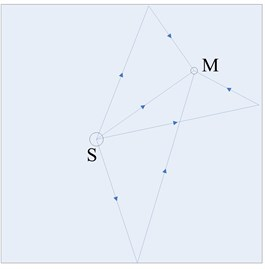As can be seen from Fig. 1:

14
$x\left(M\right)=u\left(M\right)+\sum _{i=0}^{\mathrm{\infty }}{\rho }_{i}s\left(M-i{M}_{i}\right),$

where $u\left(M\right)$ is the noise, ${\rho }_{i}$ is the Reflection coefficient, $s\left(M-i{M}_{i}\right)$ is the signal of reflection, $s\left(M\right)$ is the signal of sound source, $x\left(M\right)$ is the total signal. Ignore the noise, then Eq. (14) can express:

15
$x\left(M\right)=\sum _{i=0}^{\mathrm{\infty }}{\rho }_{i}s\left(M-i{M}_{i}\right).$

Convolution represented by the formula:

16
$x\left(M\right)=s\left(M\right)\mathrm{*}\sum _{i=0}^{\mathrm{\infty }}{\rho }_{i}\delta \left(M-i{M}_{i}\right),$
17
$x\left(M\right)=s\left(M\right)\mathrm{*}h\left(M\right),$

where $h\left(M\right)$ is impulse response of the room. From the Eq. (17), acoustic reflection can be seen as a form of noise Convolution noise. While taking the Eq. (17) on both sides do Fourier transform and logarithmic:

18
$\stackrel{^}{X}\left(\omega \right)=\stackrel{^}{S}\left(\omega \right)+\stackrel{^}{H}\left(\omega \right).$

Both sides of the equation called cepstral, design a passband filter make the $\stackrel{^}{H}\left(\omega \right)$ disappear, and make Inverse Fourier transform of $\stackrel{^}{S}\left(\omega \right)$, then we can obtain $s\left(n\right)$:

Error is defined as:

19
$error=\frac{{‖{p}_{l}\left(s\right)-{p}_{h}\left(s\right)‖}_{2}}{{‖{p}_{l}\left(s\right)‖}_{2}}×100\mathrm{}\mathrm{%}.$

## 3. Numerical simulation

In order to verify that difference of the sound signal before and after data processing, respectively, two and four-point source source reconstruction simulation have been done. Of the parameters of the simulation is the same, the enclosed space length, width and height, respectively, 1 m, 1 m, 1 m. Analysis of the frequency are 800 Hz, surface vibration velocity of the ball is 0.25 m/s, holographic measurement distance is 0.1 m, reconstructed surface distance is 0.05 m, holographic surface is 64×64 square planar array, planar array of edge length is 0.8 m. The close space impulse response is shown in Fig. 2. The characteristics of passband filter is shown in Fig. 3. Two-point and four-point source source simulation results shown in Fig. 4 to Fig. 9.

Fig. 2The close space impulse response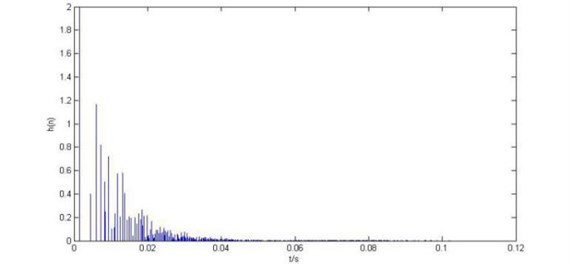Fig. 3Bandpass filter amplitude-frequency characteristics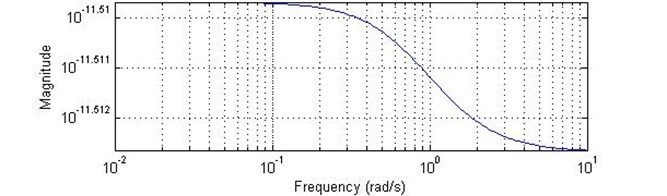Fig. 4Theoretical sound pressure contours of two sound sources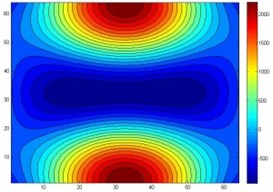Fig. 5The reconstruction of two point sources acoustic pressure contours after data processing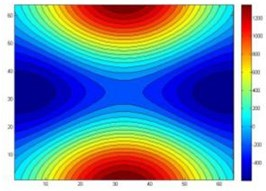Fig. 6The reconstruction of two point sources acoustic pressure contours before data processing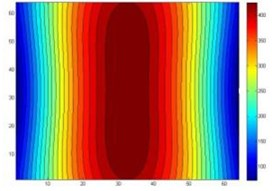Fig. 7Theoretical sound pressure contours of four sound sources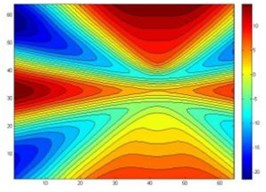Fig. 8The reconstruction of four point sources acoustic pressure contours after data processing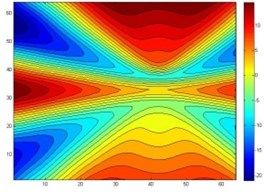Fig. 9The reconstruction of four point sources acoustic pressure contours before data processing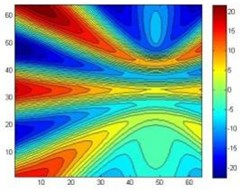As can be seen from the simulation graph, the data of the two-point sources and four-point sources after the data processing could reconstruct the sound source precisely, there will be greater reconstruction error before data processing. For two sources, the sound source overlap portion is more, and cannot distinguish between two sources. For a four-point source, the sound pressure cloud also has a relatively large distortion. Error is shown in Fig. 10.

Fig. 10Error analysis figure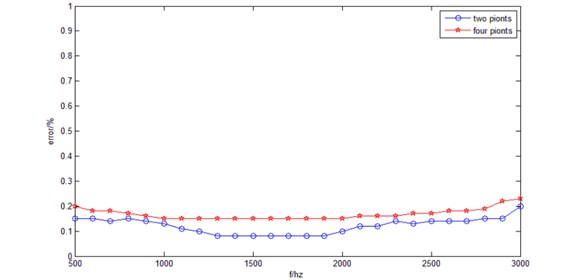From Fig. 10, in the low frequency the error can be controlled within 20 %, the error of two sources is less than the error of four-point sources. In 1300 Hz to 1900 Hz band, two sources of error control in about 8 %, but with increased frequency, the error increases. This is caused by the complex cepstrum algorithm itself restricted, optimizing the parameters or combining with other reverberation algorithms the error can be controlled within a smaller range.

## 4. Conclusions

This paper proposes a method about reconstruction of the sound source in closed space with NAH. After receiving the flat plane array sound pressure data by the method of complex cepstrum data processing, data processed planar NAH sound source reconstruction. Simulation results show that the sound pressure level is very similar to the theoretical value of the post processed reconstruction of the sound source. In low frequencies, error analysis shows that the method of processing data for complex cepstrum method based on 1300 Hz to 1900 Hz frequency error control is good. The reason of the increasing of the error is that the parameters of the complex cepstrum algorithm cannot adjust adaptive the frequency increases, optimizing the parameters or combining with other reverberation algorithms could control the error within a smaller range.